Enthalpy of solution of anhydrous copper (II) sulfate You are provided with a - sample of anhydrous copper sulfate - electronic scales - 100 mL measuring cylinder - goggles - gloves - labcoat - calorimeter - thermometer The aim of this activity is to measure the enthalpy of solution of anhydrous copper sulfate. CuSO4(s) + (aq) --> CuSO4(aq) Using a 100 mL measuring cylinder place a 100 mL of water into the calorimeter.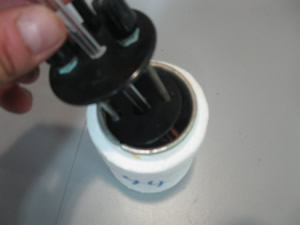Measure the temperature of the water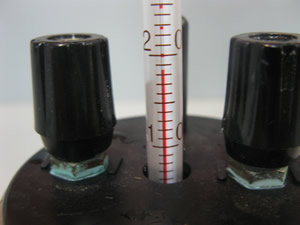Weigh accurately approximately 5.00 g of anhydrous CuSO4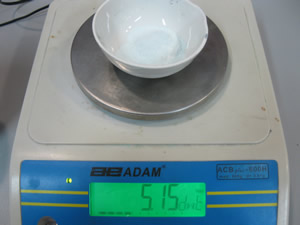Place the anhydrous copper sulfate sample into the calorimeter.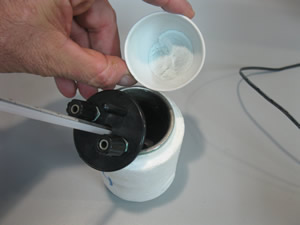Measure the highest temperature reached by the water.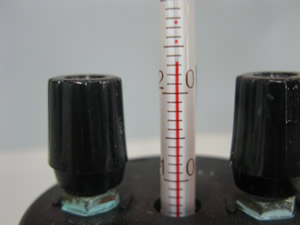Let's practice the calculations given the information above, before you calculate the ΔH using your own results..1) Calculate the amount, in mole, of anhydrous CuSO4 2) Calculate the amount of heat energy given off by this amount of anhydrous CuSO4 3) Calculate the ΔH for the reaction below. CuSO4 + (aq) ---> CuSO4(aq) ......... ΔH = ? Now calculate the ΔH for the reaction above using your results.